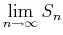Next: The Integral Test Up: lab_template Previous: Getting Started

## Background

An infinite series is the sum of an infinite sequence. More precisely, the sum of an infinite series is defined as the limit of the sequence of the partial sums of the terms of the series, provided this limit exists. If no finite limit exists, then we say that the series is divergent.

The sum of an infinite series is defined as, whereis the partial sum of the first n terms of the series. However, because of the algebraic difficulty of expressingas a function of n, it is usually not possible to find sums by directly using the definition.

So, if we can generally not work from the definition, what can be done? There are several convergence tests that provide us with some needed tools. These are tests that tell us if a series converges, but in the case that the series does converge, does not tell us the sum of the series.

The convergence tests that will be used in this lab are the integral test and the ratio test.

Dina J. Solitro-Rassias
2017-11-09